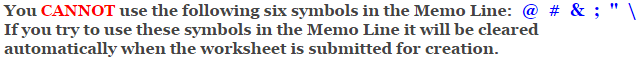# Venn Diagram Worksheets

## Set Notation Problems Using Two Sets Worksheets

This Venn Diagram Worksheet is a great for practicing solving set notation problems of different sets, unions, intersections, and complements with two sets. You may select to use single sets, unions, intersection, and relative complements of sets. This Venn Diagram Worksheet will produce three problems with a maximum of 4 questions for each Venn Diagram for the students to answer.

 Number of Possible Questions For Each Problem Type of Set Operations Standard Sets Compliments of Sets Both Types of Sets Single Sets 2 2 4 Union of Sets 1 4 5 Intersection of Sets 1 4 5 Relative Complements of Sets 2 8 10 Totals 6 18 24

The table above shows the number of possible questions that will be generated for each Venn Diagram problem, based on the selection of set operations and set types. This Venn Diagram Worksheet will create three different problems each with a maximum of four questions.

For example if you choose Single Set operations and Standard Set types, the worksheet will generate two questions for each of the three problems on the page.

If you choose Union of Sets for the operation and Both Standard and Complements of Set types, the worksheet will randomly choose 4 from the possible 5 different questions for the three problems.

### Language for the Venn Diagram Worksheet

 English German Albanian Spanish Swedish Italian French Turkish Polish Norwegian

### Memo Line for the Venn Diagram Worksheet

You may enter a message or special instruction that will appear on the bottom left corner of the Venn Diagram Worksheet.### Venn Diagram Worksheet Answer Page

Now you are ready to create your Venn Diagram Worksheet by pressing the Create Button.

 Recommended Videos

If You Experience Display Problems with Your Math Worksheet